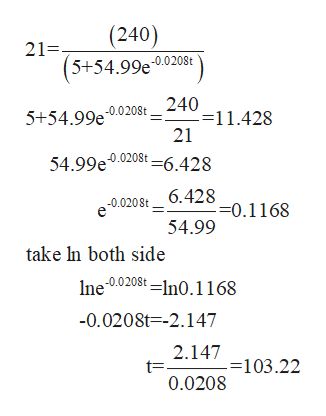# In 1990 the populations of a country was estimated at 4 million. For any year the population is modeled by this logistic equations:  P(t)= (240)/(5+54.99e -.0208t) , where is the number of years since 1990. Estimate the year that the population will be 21 million.

Question
1 views

In 1990 the populations of a country was estimated at 4 million. For any year the population is modeled by this logistic equations:  P(t)= (240)/(5+54.99e -.0208t) , where is the number of years since 1990. Estimate the year that the population will be 21 million.

check_circle

Step 1

Given information

Population of country in 1990 = 4 million

Population for any year logistic equation

Step 2

To determine

The year when population will be 21 million

Step 3

Calculation

Put p(t) = 21 in giv...help_outlineImage Transcriptionclose(240) 21=- (5+54.99e0.0208t 240 -=11.428 21 5+54.99e -0.0208t 54.99e0.0208t6.428 6.428 --0.1168 54.99 -0.020St take In both side Ine 0.0208t ln0.1168 -0.0208t -2.147 2.147 -=103.22 0.0208 fullscreen

### Want to see the full answer?

See Solution

#### Want to see this answer and more?

Solutions are written by subject experts who are available 24/7. Questions are typically answered within 1 hour.*

See Solution
*Response times may vary by subject and question.
Tagged in

### Calculus